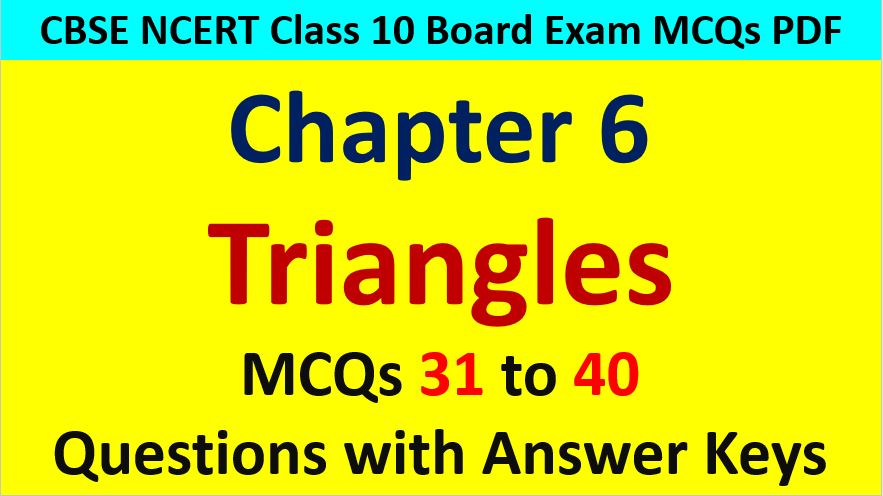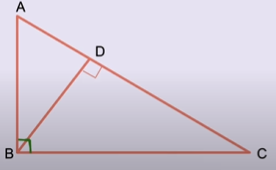Friday, October 22, 2021
Home > CBSE Class 10 > Extra MCQ Questions for Class 10 Maths Chapter 6 Triangles

# Extra MCQ Questions for Class 10 Maths Chapter 6 TrianglesHi students, Welcome to AMBiPi (Amans Maths Blogs). In this article, you will get Extra MCQ Questions for Class 10 Maths Chapter 6 Triangles. You can download this PDF and save it in your mobile device or laptop etc.

Extra MCQ Questions for Class 10 Maths Triangles Question No 31:

A 15 m high tower casts a shadow 24 m long at certain time and at the same time a telephone pole casts a shadow of 16 m long. Find the height of the telephone pole.

Option A : 10 cm

Option B : 12 cm

Option C : 15 cm

Option D : 9 cm

Option A : 10 cm

Extra MCQ Questions for Class 10 Maths Triangles Question No 32:

A 5 m long ladder is placed leaning towards a vertical wall such that it reaches the wall at a point of 4 m high. If the foot of the ladder is moved 1.6 m towards the wall, then find the distance by which the top of the ladder would slide upwards on the wall.

Option A : 0.2 m

Option B : 0.8 m

Option C : 2 m

Option D : 1.7 m

Option B : 0.8 m

Extra MCQ Questions for Class 10 Maths Triangles Question No 33:

A street light bulb is fixed on a pole 6 m above the level of the street. If a women of height 1.5 m casts a shadow of 3 m, then what is the how far she is away from the base of the pole.

Option A : 6 m

Option B : 12 m

Option C : 9 m

Option D : None of these

Option C : 9 m

Extra MCQ Questions for Class 10 Maths Triangles Question No 34:

In figure below, ABC is a right triangle with B as right angled and BD ⟂ AC. If AD = 4 cm and CD = 5 cm. Find BD and AB.Option A : √5, 6

Option B : 2√5, 5

Option C : 2√5, 6

Option D : 3√2, 5

Option C : 2√5, 6

Extra MCQ Questions for Class 10 Maths Triangles Question No 35:

In the figure below, PQR is a right angled triangle with right angled at Q and QS ⟂ PR. If PQ = 6, PS = 4, then find the values of QS, RS and QR.Option A : 5, 2√5, 3√5

Option B : 2√5, 5, 6

Option C : 2√5, 5, 3√5

Option D : 3, 4, 5

Option C : 2√5, 5, 3√5

Extra MCQ Questions for Class 10 Maths Triangles Question No 36:

In the figure below, ∆ABC is a right angled triangle and ∆ACE, ∆BCD and ∆ABF are equilateral triangles. If A1, A2, A3 are the areas of the triangles in respective regions as in figure, thenOption A : A1 = 2(A2 + A3)

Option B : A3 = (A1 + A2)

Option C : A22 = A12 + A32

Option D : None of these

Option B : A3 = (A1 + A2)

Extra MCQ Questions for Class 10 Maths Triangles Question No 37:

In the figure below, three semicircles are drawn taking AB, BC, CA as the diameter, then which of the following is true?Option A : A2 = (A1 + A3)

Option B : A3 = (A1 + A2)

Option C : A12 = A22 + A32

Option D : None of these

Option B : A3 = (A1 + A2)

Extra MCQ Questions for Class 10 Maths Triangles Question No 38:

Foot of a 10 m long ladder leaning against a vertical wall is 6 m away from the base of the ball. Then, the height of the point on the wall where the top of ladder reaches is

Option A : 6 m

Option B : 8 m

Option C : 13 m

Option D : 12 m

Option B : 8 m

Extra MCQ Questions for Class 10 Maths Triangles Question No 39:

A flag pole 18 m high casts a shadow 9.6 m long. Then, the distance of the top of the pole from the far end of the shadow is

Option A : 10.4

Option B : 11.4

Option C : 20.4

Option D : 21.4

Option C : 20.4

Extra MCQ Questions for Class 10 Maths Triangles Question No 40:

In the figure below, PA, QB, RC and SD are all perpendiculars to a line l, AB = 6 cm, BC = 9 cm, CD = 12 cm and SP = 36 cm. Find PQ, QR and RS.Option A : 8, 12, 16

Option B : 4, 3, 5

Option C : 10, 8, 6

Option D : None of these